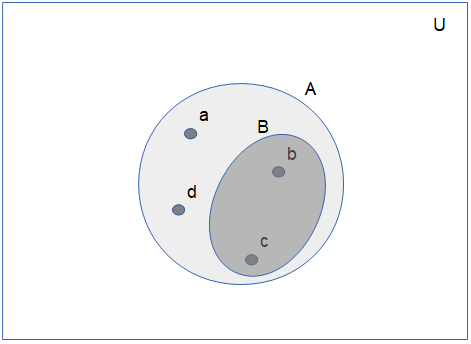Welcome guest
You're not logged in.
279 users online, thereof 0 logged in

## Definition: Subset and Superset

Let $$X$$ be a set. A set $$A$$ is called a subset of $$X$$ and denoted by $$A\subseteq X$$, if each element of $$A$$ is also an element of $$X$$. Equivalently, X is a superset of $$A$$, denoted as $$X\supseteq A$$.

If, in addition, $$A\neq X$$, then $$A$$ is called a proper subset of $$X$$ and denoted by $$A\subset X$$. Equivalently, X is then a proper superset of $$A$$, and denoted as $$X\supset A$$.

We can draw supersets and subsets as Venn diagrams. In the following figure, $B$ is a subset of $A$ and $A$ is a subset of the universal set $U$:### Examples

1. The set of all animals is the superset of all dogs.
2. The set of $A=\{\text{Italy,Georgia,Brasil}\}$ is a subset of the set $C=\{x:\, x \text{ is a country}\}.$
3. The set of $\mathbb Z$ of all integers is a subset of the set $\mathbb Q$ of all rational numbers.
4. A line on a plane is a subset of this plane.